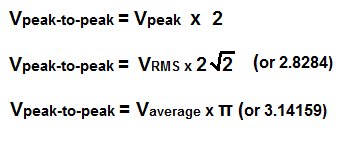﻿ Peak-to-Peak Voltage (VPP) Calculator ﻿# Peak-to-peak Voltage (VPP) CalculatorV

0.0000V

V

0.0000V

### Average to Peak-to-Peak Voltage Calculator

V

0.0000V

The Peak-to-peak Voltage calculator calculates the peak-to-peak Voltage, VP-P, value from either the peak voltage, the RMS voltage, or the average voltage. It calculates the peak-to-peak Voltage based on the above formulas for each respectively.

To compute VP-P from the peak voltage, the peak voltage is multiplied by 2.

To compute VP-P from the RMS voltage, the RMS voltage is multiplied by 2.8284.

To compute VP-P from the average voltage, the average voltage is multiplied by 3.14159.

A user enters the voltage, V and the result will automatically be calculated and shown. The peak-to-peak voltage result which is displayed above is in unit volts (V).

The peak-to-peak voltage represents the voltage from the all the way from the bottom of the waveform (called the trough) to the very top of the waveform (called the crest). It is used often to represent the amplitude of a voltage waveform. However, since there are so many other ways of specifying a voltage, including RMS voltage, average voltage and peak voltage, it should be specified as a peak-to-peak value, such as 5Vpeak-to-peak.

Related Resources

﻿